# Jacobson radical

(diff) ← Older revision | Latest revision (diff) | Newer revision → (diff)
Jump to: navigation, search

of a ringThe ideal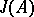of an associative ring (cf. Associative rings and algebras)which satisfies the following two requirements: 1)is the largest quasi-regular ideal in(a ringis called quasi-regular if the equation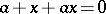is solvable for any of its elements); and 2) the quotient ringcontains no non-zero quasi-regular ideals. The radical was introduced and studied in detail in 1945 by N. Jacobson .

The Jacobson radical always exists and may be characterized in very many ways:is the intersection of the kernels of all irreducible representations of the ring; it is the intersection of all modular maximal right ideals (cf. Modular ideal); it is the intersection of all modular maximal left ideals; it contains all quasi-regular one-sided ideals; it contains all one-sided nil ideals; etc. Ifis an ideal of, then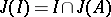. If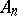is the ring of all matrices of orderover, then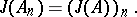If the following-composition is introduced on the associative ring: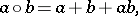then the radicalin the semi-group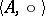will be a subgroup with respect to the composition.

There are no non-zero irreducible finitely-generated modules over a quasi-regular associative ring (i.e. an associative ring coinciding with its own Jacobson radical), but there exist simple associative quasi-regular rings. The Jacobson radical of the associative ringis zero if and only ifis a subdirect sum of primitive rings (cf. Primitive ring).